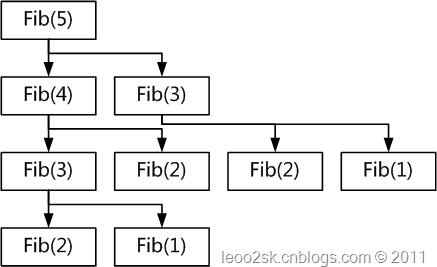#0
3
01. 云栖社区>
2. 博客>
3. 正文

## 程序设计中的计算复用(Computational Reuse)

nothingfinal 2018-03-10 19:36:00 浏览580

# 从斐波那契数列说起

 `public` `static` `ulong` `Fib(``ulong` `n)` `{` `    ``return` `(n == 1 || n == 2) ? 1 : Fib(n - 1) + Fib(n - 2);` `}`1 2 3 4 5 6 7 8 9 10 11 12 13 14 15 `public` `static` `ulong` `Fib(``ulong` `n)` `{` `    ``if` `(n == 1 || n == 2)` `    ``{` `        ``return` `1;` `    ``}` `    ``ulong` `m1 = 1, m2 = 1;` `    ``for` `(``ulong` `i = 3; i <= n; i++)` `    ``{` `        ``m2 = m1 + m2;` `        ``m1 = m2 - m1;` `    ``}`   `    ``return` `m2;` `}`

# 计算复用对软件开发的启示

 1 2 3 4 5 `foreach``(``\$items` `as` `\$k` `=> ``\$v``){` `    ``//...` `    ``\$money` `= ``\$v``->money + getTax();` `    ``//...` `}`

 1 2 3 4 5 6 `\$tax` `= getTax();` `foreach``(``\$items` `as` `\$k` `=> ``\$v``){` `    ``//...` `    ``\$money` `= ``\$v``->money + ``\$tax``;` `    ``//...` `}`

 1 `echo` `count``(``\$arr``);`

nothingfinal
+ 关注